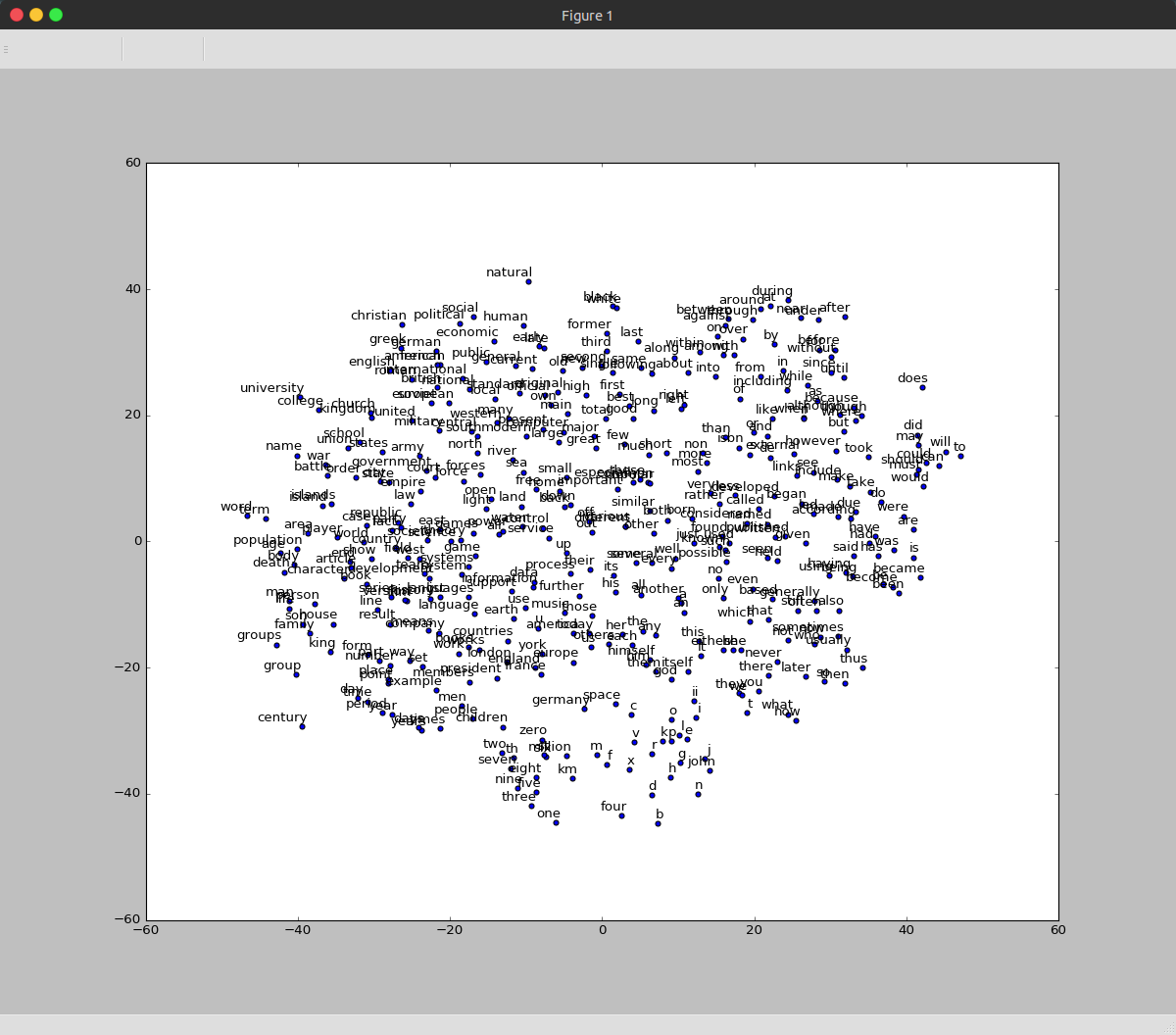# TensorFlow深度学习笔记 循环神经网络实践 - 梦里风林 - 博客园Github工程地址：https://github.com/ahangchen/GDLnotes

## 加载数据

• 使用text8作为训练的文本数据集

text8中只包含27种字符：小写的从a到z，以及空格符。如果把它打出来，读起来就像是去掉了所有标点的wikipedia。

• 用zipfile读取zip内容为字符串，并拆分成单词list
• 用connections模块统计单词数量并找出最常见的单词

## 构造计算单元

``````embeddings = tf.Variable(
tf.random_uniform([vocabulary_size, embedding_size], -1.0, 1.0))``````
• 构造一个vocabulary_size x embedding_size的矩阵，作为embeddings容器，
• 有vocabulary_size个容量为embedding_size的向量，每个向量代表一个vocabulary，
• 每个向量的中的分量的值都在-1到1之间随机分布
``embed = tf.nn.embedding_lookup(embeddings, train_dataset)``
• 调用tf.nn.embedding_lookup，索引与train_dataset对应的向量，相当于用train_dataset作为一个id，去检索矩阵中与这个id对应的embedding
``````loss = tf.reduce_mean(
tf.nn.sampled_softmax_loss(softmax_weights, softmax_biases, embed,
train_labels, num_sampled, vocabulary_size))``````
• 采样计算训练损失
``optimizer = tf.train.AdagradOptimizer(1.0).minimize(loss)``
• 自适应梯度调节器，调节embedding列表的数据，使得偏差最小
• 预测，并用cos值计算预测向量与实际数据的夹角作为预测准确度（相似度）指标

## 传入数据进行训练

• 切割数据用于训练，其中：
``data_index = (data_index + 1) % len(data)``
• 依旧是每次取一部分随机数据传入
• 等距离截取一小段文本
• 构造训练集：每个截取窗口的中间位置作为一个train_data
• 构造标签：每个截取窗口中，除了train_data之外的部分，随机取几个成为一个list，作为label（这里只随机取了一个）
• 这样就形成了根据目标词汇预测上下文的机制，即Skip-gram
• 训练100001次，每2000次输出这两千次的平均损失
• 每10000次计算相似度，并输出与验证集中的词最接近的词汇列表
• 用tSNE降维呈现词汇接近程度
• 用matplotlib绘制结果## CBOW

• 修改截取数据的方式
• 构造标签：每个截取窗口的中间位置作为一个train_label
• 构造训练集：每个截取窗口中，除了train_label之外的部分，作为train_data（这里只随机取了一个）
• 这样就形成了根据上下文预测目标词汇的机制，即CBOW
• 分别从embeding里找到train_data里每个word对应的vector，用tf.reduce_sum将其相加，将相加结果与train_label比较
``````# Look up embeddings for inputs.
embed = tf.nn.embedding_lookup(embeddings, train_dataset)
# sum up vectors on first dimensions, as context vectors
embed_sum = tf.reduce_sum(embed, 0)``````
• 训练中依旧是调节embeding的参数来优化loss
• 训练结果如下图，可以看到不同单词的接近程度cbow.py

## RNN 造句

### 训练数据

• BatchGenerator
• text: 全部的文本数据
• text_size：全部文本的字符串长度
• batch_size：每段训练数据的大小
• num_unrollings：要生成的训练数据段的数目
• segment：整个训练数据集可以分成几个训练数据片段
• cursor：重要，
• 一开始记录每个训练数据片段的起始位置坐标，即这个片段位于text的哪个index
• 执行next_batch生成一个训练数据的时候，游标会从初始位置自增，直到取够batch_size个数据
• last_batch：上一个训练数据片段
• 每调用一次next，生成一个num_unrollings长的array，以last_batch开头，跟着num_unrollings个batch
• 每个batch的作为train_input，每个batch后面的一个batch作为train_label，每个step训练num_unrolling个batch

### lstm-cell

• 为了解决消失的梯度问题，引入lstm-cell，增强model的记忆能力
• 根据这篇论文设计lstm-cell: http://arxiv.org/pdf/1402.1128v1.pdf
• 分别有三个门：输入门，遗忘门，输出门，构成一个cell
• 输入数据是num_nodes个词，可能有vocabulary_size种词
• 输入门：
``  input_gate = sigmoid(i * ix + o * im + ib)``
``````- 给输入乘一个vocabulary_size * num_nodes大小的矩阵，给输出乘一个num_nodes * num_nodes大小的矩阵;
- 用这两个矩阵调节对输入数据的取舍程度
- 用sigmoid这个非线性函数进行激活``````
• 遗忘门：
``  forget_gate = sigmoid(i * fx + o * fm + fb)``

• 输出门：
``  output_gate = sigmoid(i * ox + o * om + ob)``

• 组合：
``````  update = i * cx + o * cm + cb
state = forget_gate * state + input_gate * tanh(update)
lstm_cell = output_gate * tanh(state)``````
``````- 用同样的方式构造新状态update
- 用遗忘门处理历史状态state
- 用tanh激活新状态update
- 用输入门处理新状态update
- 整合新旧状态，再用tanh激活状态state
- 用输出门处理state``````

### lstm优化

``````values = tf.split(1, gate_count, tf.matmul(i, input_weights) + tf.matmul(o, output_weights) + bias)
input_gate = tf.sigmoid(values)
forget_gate = tf.sigmoid(values)
update = values``````

### Optimizer

• 采用one-hot encoding作为label预测
• 采用交叉熵计算损失
• 引入learning rate decay

### Flow

• 填入训练数据到placeholder中
• 验证集的准确性用logprob来计算，即对可能性取对数
• 每10次训练随机挑取5个字母作为起始词，进行造句测试
• 你可能注意到输出的sentence是由sample得到的词组成的，而非选择概率最高的词，这是因为，如果一直取概率最高的词，最后会一直重复这个概率最高的词

## Embedding look up

• 数据输入：BatchGenerator不再生成one-hot-encoding的向量作为输入，而是直接生成bigram对应的index列表
• embedding look up调整embedding，使bigram与vector对应起来
• 将embedding look up的结果喂给lstm cell即可
• 输出时，需要将label和output都转为One-hot-encoding，才能用交叉熵和softmax计算损失
• 在tensor里做data到one-hot-encoding转换时，主要依赖tf.gather函数
• 在对valid数据做转换时，主要依赖one_hot_voc函数

## Drop out

• 在lstm cell中对input和output做drop out

## Seq2Seq

• 最后一个问题是，将一个句子中每个词转为它的逆序字符串，也就是一个seq到seq的转换
• 正经的实现思路是，word 2 vector 2 lstm 2 vector 2 word
• 不过tensorflow已经有了这样一个模型来做这件事情：Seq2SeqModel，关于这个模型可以看这个分析
以及tensorflow的example
• 只需要从batch中，根据字符串逆序的规律生成target sequence，放到seq2seqmodel里即可，主要依赖rev_id函数
• 实现见seq2seq.py
• 注意，用Seq2SeqModel的时候，size和num_layer会在学习到正确的规律前就收敛，我把它调大了一点
``````def create_model(sess, forward_only):
model = seq2seq_model.Seq2SeqModel(source_vocab_size=vocabulary_size,
target_vocab_size=vocabulary_size,
buckets=[(20, 21)],
size=256,
num_layers=4,
batch_size=batch_size,
learning_rate=1.0,
learning_rate_decay_factor=0.9,
use_lstm=True,
forward_only=forward_only)
return model``````
• 参数含义
• source_vocab_size: size of the source vocabulary.
• target_vocab_size: size of the target vocabulary.
• buckets: a list of pairs (I, O), where I specifies maximum input length
that will be processed in that bucket, and O specifies maximum output
length. Training instances that have inputs longer than I or outputs
longer than O will be pushed to the next bucket and padded accordingly.
We assume that the list is sorted, e.g., [(2, 4), (8, 16)].
• size: number of units in each layer of the model.
• num_layers: number of layers in the model.
• max_gradient_norm: gradients will be clipped to maximally this norm.
• batch_size: the size of the batches used during training;
the model construction is independent of batch_size, so it can be
changed after initialization if this is convenient, e.g., for decoding.
• learning_rate: learning rate to start with.
• learning_rate_decay_factor: decay learning rate by this much when needed.
• use_lstm: if true, we use LSTM cells instead of GRU cells.
• num_samples: number of samples for sampled softmax.
• forward_only: if set, we do not construct the backward pass in the model.

## 参考链接

### 觉得文章有用就打赏一下文章作者

#### 支付宝扫一扫打赏#### 微信扫一扫打赏• QQ咨询
• 回顶